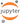Exploring Multiple Variables in EDA

In this section, we will continue re-using the data from the previous post based on Pseudo Facebook data from udacity.

The data from the project corresponds to a typical data set at Facebook. You can load the data through the following command. Notice that this is a TAB delimited tsv file. This data set consists of 99000 rows of data. We will see the details of different columns using the command below.

In :
import pandas as pd
import numpy as np
cats = ['userid', 'dobday', 'dobyear', 'dob_month']
for col in pf.columns:
if col in cats:
pf[col] = pf[col].astype('category')
#summarize data
pf.describe(include='all', percentiles=[]).T.replace(np.nan,' ', regex=True)

/usr/lib/python3.5/site-packages/numpy/lib/function_base.py:3834: RuntimeWarning: Invalid value encountered in percentile
RuntimeWarning)

Out:
count unique top freq mean std min 50% max
userid 99003.0 99003 2.19354e+06 1
age 99003.0 37.2802 22.5897 13 28 113
dob_day 99003.0 31 1 7900
dob_year 99003.0 101 1995 5196
dob_month 99003.0 12 1 11772
gender 98828.0 2 male 58574
tenure 99001.0 537.887 457.65 0 3139
friend_count 99003.0 196.351 387.304 0 82 4923
friendships_initiated 99003.0 107.452 188.787 0 46 4144
likes 99003.0 156.079 572.281 0 11 25111
likes_received 99003.0 142.689 1387.92 0 8 261197
mobile_likes 99003.0 106.116 445.253 0 4 25111
mobile_likes_received 99003.0 84.1205 839.889 0 4 138561
www_likes 99003.0 49.9624 285.56 0 0 14865
www_likes_received 99003.0 58.5688 601.416 0 2 129953

Continuing with our analysis from the last post, finding a relationship between age and friends counts, let us add gender to the equation.

In order to do this, we will first use groupby() and agg() to get group by data.

In :
def groupByStats(df, groupCol, statsCol):
''' return a dataframe with groupByCo
groupbyCol: a string or a list of strings for col names in df
statsCol: a string for col in df of which we need stats for
'''

# Define the aggregation calculations
aggregations = {
statsCol: {
(statsCol+'_mean'): 'mean',
(statsCol+'_median'): 'median',
(statsCol+'_q25'): lambda x: np.percentile(x,25),
(statsCol+'_q75'): lambda x: np.percentile(x,75),
'n': 'count'
}
}
df_group_by_groupCol = df.groupby(groupCol, as_index=False, group_keys=False).agg(aggregations)
df_group_by_groupCol.columns = df_group_by_groupCol.columns.droplevel()
if isinstance(groupCol, list):
cols = groupCol + list(df_group_by_groupCol.columns)[len(groupCol):]
else:
cols = [groupCol] + list(df_group_by_groupCol.columns)[1:]
df_group_by_groupCol.columns = cols
return df_group_by_groupCol

In :
pf_group_by_age_gender = groupByStats(pf[pf.gender.notnull()], ['gender', 'age'], 'friend_count')

Out:
gender age friend_count_q75 n friend_count_mean friend_count_median friend_count_q25
0 female 13 316.00 193 259.160622 148.0 39.0
1 female 14 410.50 847 362.428571 224.0 79.0
2 female 15 592.50 1139 538.681299 276.0 104.0
3 female 16 581.25 1238 519.514540 258.5 102.0
4 female 17 586.75 1236 538.994337 245.5 81.0

Now, we can use plots to compare friend counts across age and gender.

In :
import matplotlib.pyplot as plt
import seaborn as sns
sns.set(style="darkgrid")
%matplotlib inline
pfgroupbyagegender['unit'] = "U"
ax = sns.tsplot(time='age', value='friendcountmedian', condition='gender', unit='unit', data=pfgroupbyagegender)
plt.ylabel("Friend Count Median")
plt.xlim(10,113)

Out:
(10, 113)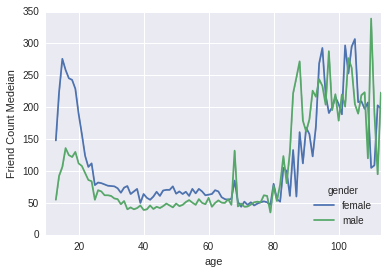It would be helpful to analyze these differences in relative terms. So lets answer a different question - how many times more friends does the average female users have than average male users.

To answer the above question we will need to transform our data. Right now, our data is in “long format” - a row of data different values of different variables. We will need to convert this to a “wide format” - where we will create columns called 'male' and 'female', that will have median counts in them.

This can be done using the “pivot()” method of pandas.

In :
pf_group_by_age_gender_wide = pf_group_by_age_gender.pivot(index='age', columns='gender', values='friend_count_median')
pf_group_by_age_gender_wide.columns.name = ''
pf_group_by_age_gender_wide.reset_index(level=0, inplace=True)

Out:
age female male
0 13 148.0 55.0
1 14 224.0 92.5
2 15 276.0 106.5
3 16 258.5 136.0
4 17 245.5 125.0

Similarly, we can use the melt() function to convert a wide format data back to a long format data. Now lets plot ratio of female to male median friend counts.

In :
pf_group_by_age_gender_wide['female_male_ratio'] = pf_group_by_age_gender_wide.female/pf_group_by_age_gender_wide.male
pf_group_by_age_gender_wide['unit'] = "u"
ax = sns.tsplot(time='age', value='female_male_ratio', unit='unit', data=pf_group_by_age_gender_wide)
plt.plot([13, 113], [1, 1], linewidth=2, color='r', linestyle='--')
plt.ylabel("Female-Male Ratio")

Out:
<matplotlib.text.Text at 0x7fe86804b4a8>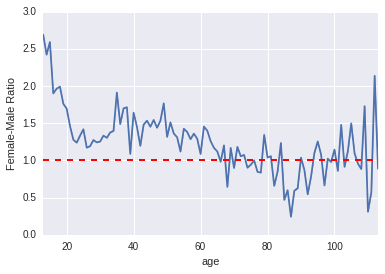In this particular case of looking at multiple variables, it would make more sense to look at count of friends as function of tenure of Facebook as well. People with a longer tenure of Facebook account are likely to accumulate a larger number of friends.

In the following section we will look at friend count of females, males at different ages along with their Facebook tenure.

In :
pf['year_joined'] = np.floor(2014 - pf['tenure']/365)

In :
pf.year_joined.value_counts(dropna=False)

Out:
 2013.0    43588
2012.0    33366
2011.0     9860
2010.0     5448
2009.0     4557
2008.0     1507
2007.0      581
2014.0       70
2006.0       15
2005.0        9
NaN            2
Name: year_joined, dtype: int64

The tabular view of this shows the distribution. We will next use the cut() method to bin this variable.

In :
pf['year_joined'] = pd.cut(pf.year_joined, bins=[2004,2009,2011,2012,2014])
pf['year_joined'] = pf['year_joined'].astype('category')

In :
pf['year_joined'].value_counts(dropna=False)

Out:
(2012, 2014]    43658
(2011, 2012]    33366
(2009, 2011]    15308
(2004, 2009]     6669
NaN                 2
Name: year_joined, dtype: int64

Let us now plot all these variables together. In particular, we want to create a plot of friend counts vs age where a different line is shown for each bin of year joined.

In :
pf_group_by_age = groupByStats(pf, ['age', 'year_joined'], 'friend_count')

Out:
age year_joined friend_count_q75 n friend_count_mean friend_count_median friend_count_q25
0 13 (2009, 2011] 691.50 11 469.818182 362.0 124.50
1 13 (2011, 2012] 406.75 48 352.333333 248.5 145.25
2 13 (2012, 2014] 167.00 425 135.668235 63.0 18.00
3 14 (2009, 2011] 1033.75 28 860.928571 517.0 279.25
4 14 (2011, 2012] 383.00 449 350.812918 224.0 109.00
In :
pf_group_by_age['unit'] = 'U'
fig, ax = plt.subplots(figsize=(8,6))
g = sns.tsplot(time='age', value='friend_count_median', unit='unit',\
condition='year_joined', data=pf_group_by_age, ax=ax)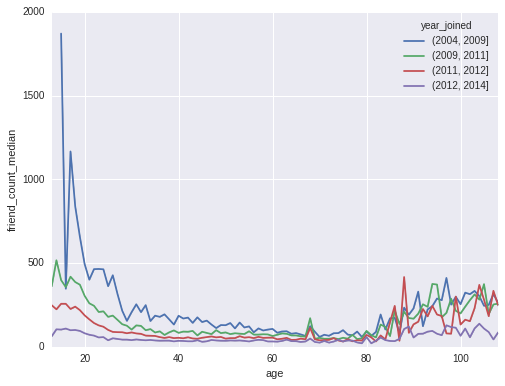Our initial hypothesis seems to be correct here - People with larger Facebook tenure tend to have higher friend counts. Lets us plot the mean of these bins and also the grand means of data.

In :
fig, ax = plt.subplots(figsize=(8,6))
g = sns.tsplot(time='age', value='friend_count_mean', unit='unit',\
condition='year_joined', data=pf_group_by_age, ax=ax)
g = pf_group_by_age.groupby('age', as_index=False).mean()
g = g.plot(x='age', y='friend_count_mean', style='--', ax=ax)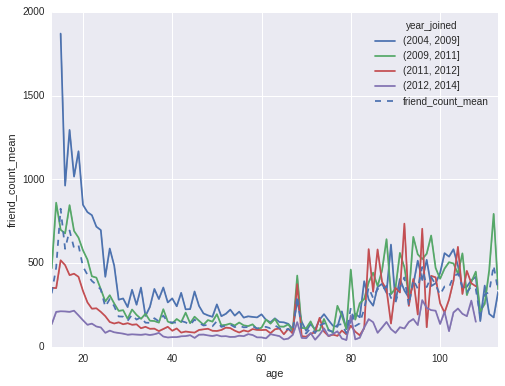Since the trend holds up after conditioning on the each of the buckets of year joined, we can increase our confidence that this observation isn’t just an artifact. Let us look at this from a different angle.

We can define a variable called “friend rate”, i.e. number of friends each person had on a per day basis.

In :
df = pf.loc[pf['tenure'] >= 1]
(df['friend_count']/df['tenure']).describe()

Out:
count    98931.000000
mean         0.609609
std          2.557356
min          0.000000
25%          0.077486
50%          0.220486
75%          0.565802
max        417.000000
dtype: float64

We can now look at the effect of this variable in more detail using the following plot:

In :
df.is_copy = False
s = df['friendships_initiated']/df['tenure']
df['friendships_initiated_per_tenure'] = s.astype('int64')
g = sns.lmplot(x="tenure", y="friendshipsinitiatedpertenure", hue="yearjoined", legendout=False,
data=df, size=5, fitreg=False, scatter_kws={'alpha': 0.5})
plt.xlim(1,3400)

Out:
(1, 3400)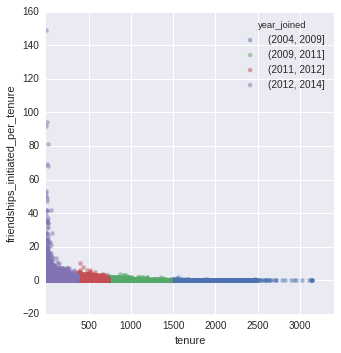This shows that people initiate less number of friends as they have longer tenure at Facebook.

Till now, we have looked at different aspects of the Facebook data set. We will now use a new data set for some more detailed multivariate analysis, and then finally come back to the Facebook data set for comparison.

We are going to work with a data set describing household purchases of five flavors of Dannon Yogurt in 8 oz sizes. Their price is recorded with each purchase occasion. This yogurt data set has a quite different structure than our pseudo-Facebook data set. The synthetic Facebook data has one row per individual with that row giving their characteristics and counts of behaviors over a single period of time. On the other hand, the yogurt data has many rows per household, one for each purchase occasion. This kind of micro-data is often useful for answering different types of questions than we’ve looked at so far.

We will start by loading the yogurt data set and then looking at its summary.

In :
yo = pd.read_csv("https://s3.amazonaws.com/udacity-hosted-downloads/ud651/yogurt.csv")
#summarize data
yo.describe(include='all', percentiles=[]).T.replace(np.nan,' ', regex=True)

Out:
count mean std min 50% max
obs 2380.0 1.367797e+03 790.076032 1.0 1369.50 2743.00
id 2380.0 2.128592e+06 17799.723643 2100081.0 2126532.00 2170639.00
time 2380.0 1.004967e+04 227.079811 9662.0 10045.00 10459.00
strawberry 2380.0 6.491597e-01 1.058612 0.0 0.00 11.00
blueberry 2380.0 3.571429e-01 0.819690 0.0 0.00 12.00
pina.colada 2380.0 3.584034e-01 0.803858 0.0 0.00 10.00
plain 2380.0 2.176471e-01 0.606556 0.0 0.00 6.00
mixed.berry 2380.0 3.886555e-01 0.904311 0.0 0.00 8.00
price 2380.0 5.925089e+01 10.913256 20.0 65.04 68.96

We notice that most of the variables are integers here. We should convert the id variable to factor. This will come handy later since the same household data is available in multiple rows.

In :
yo['id'] = yo['id'].astype('category')
#summarize data
yo.describe(include='all', percentiles=[]).T.replace(np.nan,' ', regex=True)

Out:
count unique top freq mean std min 50% max
obs 2380.0 1367.8 790.076 1 1369.5 2743
id 2380.0 332 2.13229e+06 74
time 2380.0 10049.7 227.08 9662 10045 10459
strawberry 2380.0 0.64916 1.05861 0 0 11
blueberry 2380.0 0.357143 0.81969 0 0 12
pina.colada 2380.0 0.358403 0.803858 0 0 10
plain 2380.0 0.217647 0.606556 0 0 6
mixed.berry 2380.0 0.388655 0.904311 0 0 8
price 2380.0 59.2509 10.9133 20 65.04 68.96

Let us look at the histogram of yogurt prices first.

In :
ax = sns.distplot(yo["price"], kde=False, bins=36)
plt.xlabel('Price', fontsize=12)
plt.ylabel('Count', fontsize=12)

Out:
<matplotlib.text.Text at 0x7fe8665c31d0>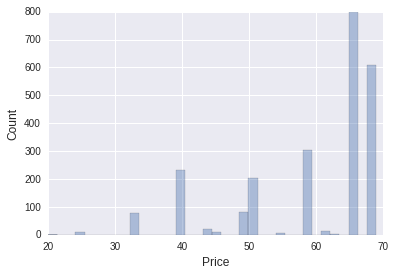Now that we have some idea about distribution of prices, let’s figure out on a given purchase occasion how many eight ounce yogurts does a household purchase. To answer this we need to combine counts of the different yogurt flavors into one variable. Then we can look at the histogram.

In :
yo['all_purchase'] = yo['strawberry']+yo['blueberry']+yo['plain']+yo['pina.colada']+yo['mixed.berry']
ax = sns.distplot(yo['all_purchase'], kde=False, bins=36)
plt.xlim(1,22)
plt.xlabel('All Purchases', fontsize=12)
plt.ylabel('Count', fontsize=12)

Out:
<matplotlib.text.Text at 0x7fe86657a198>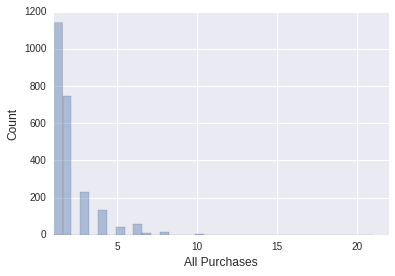It seems most household purchase one 8 oz yogurt at any given purchase. Now we will look at the scatter plot of prime vs time data.

In :
fig, ax = plt.subplots(figsize=(8,6))
sd = {'alpha': 0.25, 'edgecolors': 'black'}
g = sns.regplot(x='time', y='price', data=yo, x_jitter=2, y_jitter=0.5, \
fit_reg=False, ax=ax, color='red', scatter_kws=sd)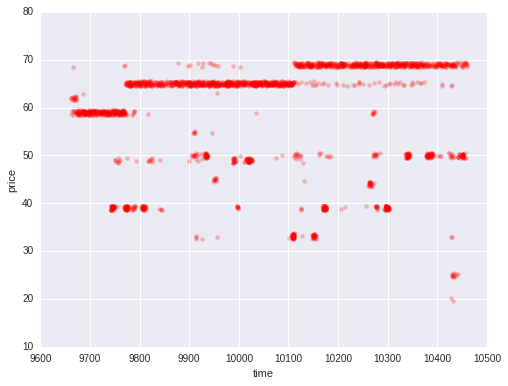We see the baseline price of yogurt has been increasing over time. We also see some scattered prices around the baseline price, which could be simply due to usage of coupons, sales etc. by customers.

When familiarizing yourself with a new data set that contains multiple observations of the same units, it’s often useful to work with a sample of those units so that it’s easy to display the raw data for that sample. In the case of the yogurt data set, we might want to look at a small sample of households in more detail so that we know what kind of within and between household variation we are working with. This analysis of a sub-sample might come before trying to use within household variation as part of a model. For example, this data set was originally used to model consumer preferences for variety. But, before doing that, we’d want to look at how often we observe households buying yogurt, how often they buy multiple items, and what prices they’re buying yogurt at. One way to do this is to look at some sub-sample in more detail. Let’s pick 16 households at random and take a closer look.

In :
sample_ids = yo.id.unique()
sample_ids = pd.Series(sample_ids).sample(16, random_state=200)
df = yo.ix[yo['id'].isin(list(sample_ids)),['id', 'price', 'time', 'all_purchase']]
df = df.reset_index(drop=True)
df['id']=pd.Series(list(df.id)).astype('category')
df.describe(include='all', percentiles=[]).T.replace(np.nan,' ', regex=True)

Out:
count unique top freq mean std min 50% max
id 171.0 16 2.13229e+06 74
price 171.0 59.8746 9.82084 33.04 65.04 68.96
time 171.0 10002.6 233.708 9664 9981 10457
all_purchase 171.0 1.84795 1.38489 1 1 10
In :
g = sns.lmplot(x='time',y='price', hue='all_purchase', data=df, scatter_kws={"s": 20*df['all_purchase']},\
col='id', col_wrap=4, size=2, aspect=1, fit_reg=False, palette="Set1")
g.set_xticklabels(rotation=90)

Out:
<seaborn.axisgrid.FacetGrid at 0x7fe866497a90>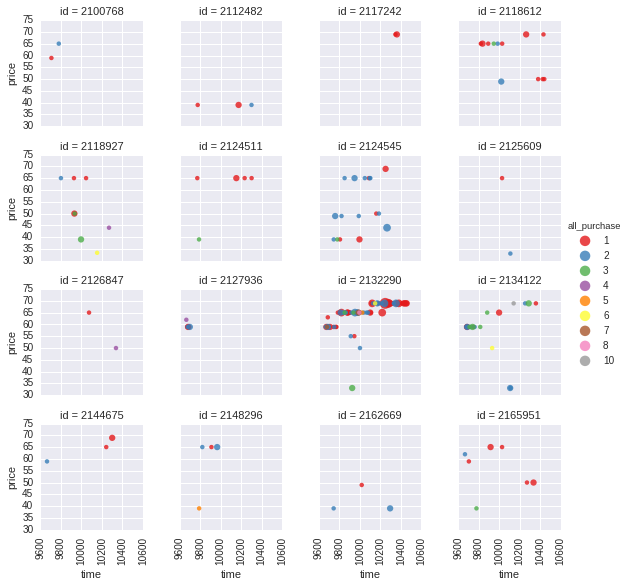From these plots, we can see the variation and how often each household buys yogurt. And it seems that some household purchases more quantities than others with the larger circles. For most of the households, the price of yogurt holds steady, or tends to increase over time.

Now, there are, of course, some exceptions, like in household 2124545 and in 2118927 household, we might think that the household is using coupons to drive the price down. Now, we don’t have the coupon data to associate with this buying data, but we can see how that information could be paired to this data to better understand the consumer behavior.

The general idea is that if we have observations over time, we can facet by the primary unit, case, or individual in the data set. For our yogurt data it was the households we were faceting over.

This faceted time series plot is something we can’t generate with our pseudo Facebook data set. Since we don’t have data on our sample of users over time.

The Facebook data isn’t great for examining the process of friending over time. The data set is just a cross section, it’s just one snapshot at a fixed point that tells us the characteristics of individuals. Not the individuals over, say, a year.

But if we had a dataset like the yogurt one, we would be able to track friendships initiated over time and compare that with tenure. This would give us better evidence to explain the difference or the drop in friendships initiated over time as tenure increases.

Much of the analysis we’ve done so far focused on some pre-chosen variable, relationship or question of interest. We then used EDA to let those chosen variables speak and surprise us. Most recently, when analyzing the relationship between two variables we look to incorporate more variables in the analysis to improve it. For example, by seeing whether a particular relationship is consistent across values of those other variables. In choosing a third or fourth variable to plot we relied on our domain knowledge. But often, we might want visualizations or summaries to help us identify such auxiliary variables. In some analyses, we may plan to make use of a large number of variables. Perhaps, we are planning on predicting one variable with ten, 20, or hundreds of others. Or maybe we want to summarize a large set of variables into a smaller set of dimensions. Or perhaps, we’re looking for interesting relationships among a large set of variables. In such cases, we can help speed up our exploratory data analysis by producing many plots or comparisons at once. This could be one way to let the data set as a whole speak in part by drawing our attention to variables we didn’t have a preexisting interest in.

We should let the data speak to determine variables of interest. There’s a tool that we can use to create a number of scatter plots automatically.

It’s called a scatter plot matrix. In a scatter plot matrix. There’s a grid of scatter plots between every pair of variables. As we’ve seen, scatter plots are great, but not necessarily suited for all types of variables. For example, categorical ones. So there are other types of visualizations that can be created instead of scatter plots. Like box plots or histograms when the variables are categorical.

Let’s produce the scatter plot matrix for our pseudo Facebook data set. We’re going to use the pairplot() method to do so.

In :
pf_subset = pf.iloc[:,1:4]
pf_subset['gender'] = pf.gender
g = sns.pairplot(data=pf_subset.sample(1000), diag_kind='kde', hue='gender')
g = g.map_diag(sns.distplot)
for ax in g.axes.flat:
plt.setp(ax.get_xticklabels(), rotation=90)

/usr/lib/python3.5/site-packages/statsmodels/nonparametric/kdetools.py:20: VisibleDeprecationWarning: using a non-integer number instead of an integer will result in an error in the future
y = X[:m/2+1] + np.r_[0,X[m/2+1:],0]*1j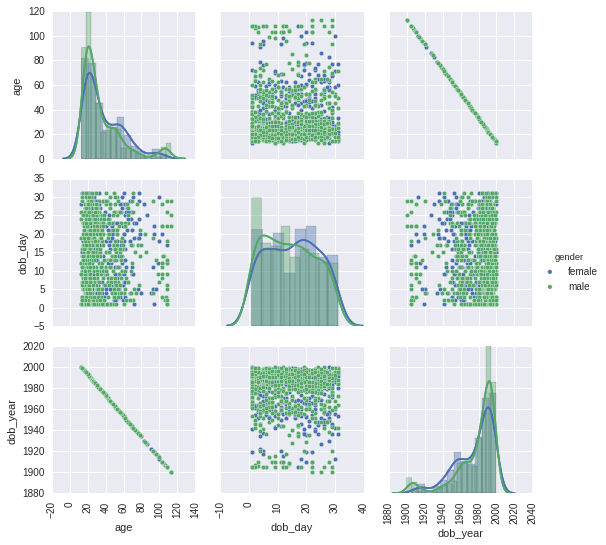In :
pf_subset = pf.iloc[:,4:8]
pf_subset['gender'] = pf.gender
g = sns.pairplot(data=pf_subset.sample(1000), diag_kind='kde', hue='gender')
g = g.map_diag(sns.distplot)
for ax in g.axes.flat:
plt.setp(ax.get_xticklabels(), rotation=90)

/usr/lib/python3.5/site-packages/statsmodels/nonparametric/kdetools.py:20: VisibleDeprecationWarning: using a non-integer number instead of an integer will result in an error in the future
y = X[:m/2+1] + np.r_[0,X[m/2+1:],0]*1j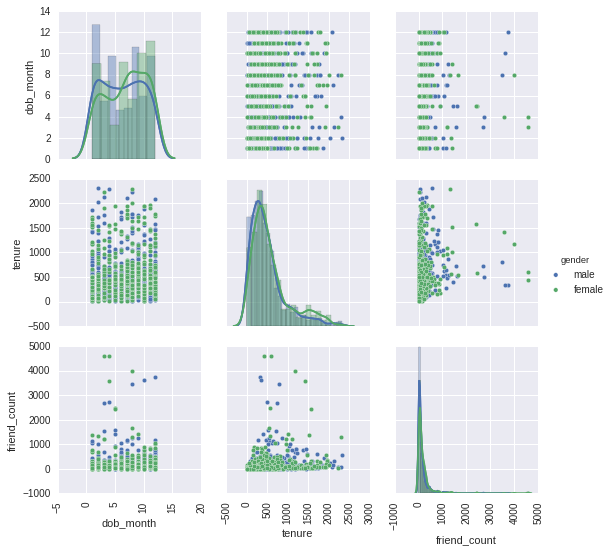In :
pf_subset = pf.iloc[:,8:11]
pf_subset['gender'] = pf.gender
g = sns.pairplot(data=pf_subset.sample(1000), diag_kind='kde', hue='gender')
g = g.map_diag(sns.distplot)
for ax in g.axes.flat:
plt.setp(ax.get_xticklabels(), rotation=90)

/usr/lib/python3.5/site-packages/statsmodels/nonparametric/kdetools.py:20: VisibleDeprecationWarning: using a non-integer number instead of an integer will result in an error in the future
y = X[:m/2+1] + np.r_[0,X[m/2+1:],0]*1j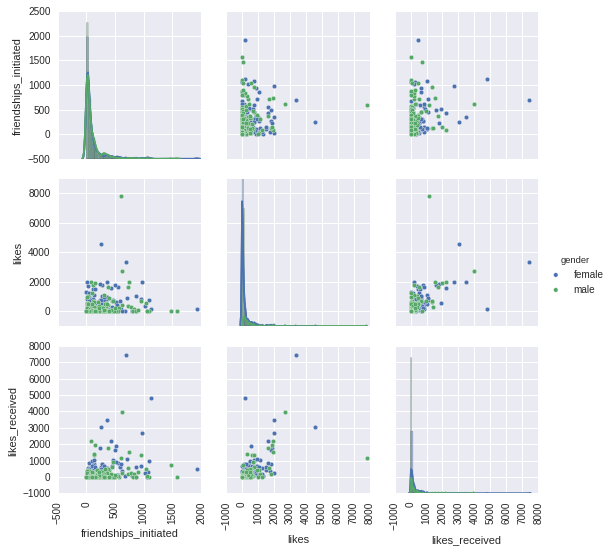In :
pf_subset = pf.iloc[:,11:-1]
pf_subset['gender'] = pf.gender
g = sns.pairplot(data=pf_subset.sample(1000), diag_kind='kde', hue='gender')
g = g.map_diag(sns.distplot)
for ax in g.axes.flat:
plt.setp(ax.get_xticklabels(), rotation=90)

/usr/lib/python3.5/site-packages/statsmodels/nonparametric/kdetools.py:20: VisibleDeprecationWarning: using a non-integer number instead of an integer will result in an error in the future
y = X[:m/2+1] + np.r_[0,X[m/2+1:],0]*1j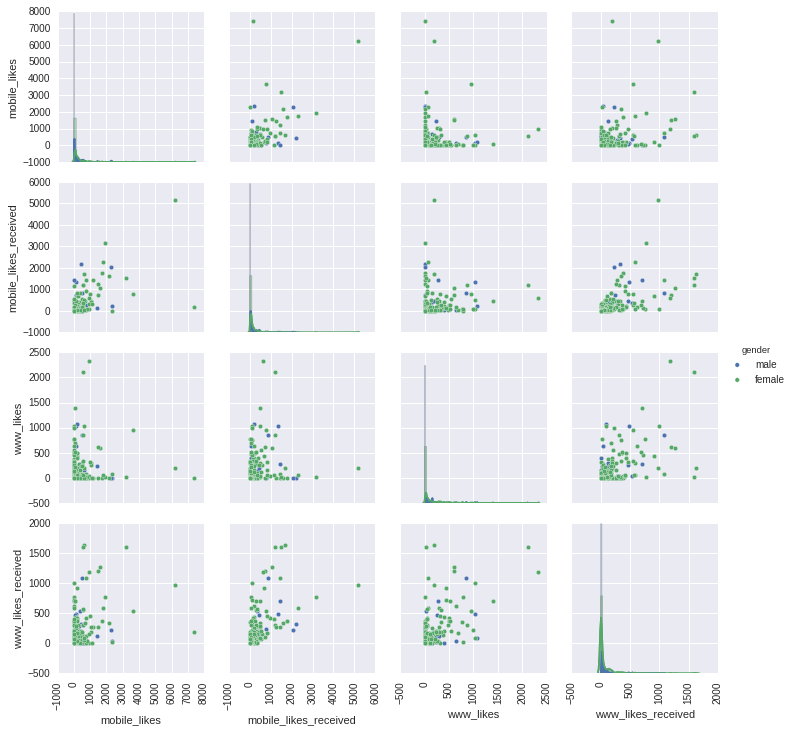At the very least, a scatter plot matrix can be a useful starting point in many analyses.

A matrix such as this one will be extremely helpful when we have even more variables than those in the pseudo-Facebook data set.

Examples arise in many areas, but one that has attracted the attention of statisticians is genomic data. In these data sets, they’re often thousands of genetic measurements for each of a small number of samples. In some cases, some of these samples have a disease, and so we’d like to identify genes that are associated with the disease.

We will use an example data set of gene expression in tumors. The data contains the expression of 6,830 genes, compared with a larger baseline reference sample.

In :
nci = pd.read_csv("https://s3.amazonaws.com/udacity-hosted-downloads/ud651/nci.tsv", sep = '\s+', header=None)
nci.columns = list(range(1,65))

In :
fig, ax = plt.subplots(figsize=(8,6))
sns.heatmap(data=nci.loc[1:200,:], vmin=0, vmax=1.0, xticklabels=False, yticklabels=False, ax=ax, cmap="YlGnBu")
plt.xlabel("Case", fontsize=14)
_ = plt.ylabel("Gene", fontsize=14)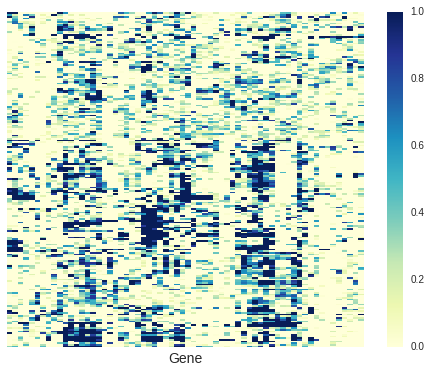Heat maps can also be a good way to look at distributions of large dimensional data.

In summary in this post, we started with simple extensions to the scatter plot, and plots of conditional summaries that you worked with in lesson four, such as adding summaries for multiple groups. Then, we tried some techniques for examining a large number of variables at once, such as scatter-plot matrices and heat maps. We also learned how to reshape data, moving from broad data with one row per case, to aggregate data with one row per combination of variables, and we moved back and forth between long and wide formats for our data.

In the next and the final post in this series, We will do an in-depth analysis of the US department of Education dataset on college education, highlighting the role of EDA.